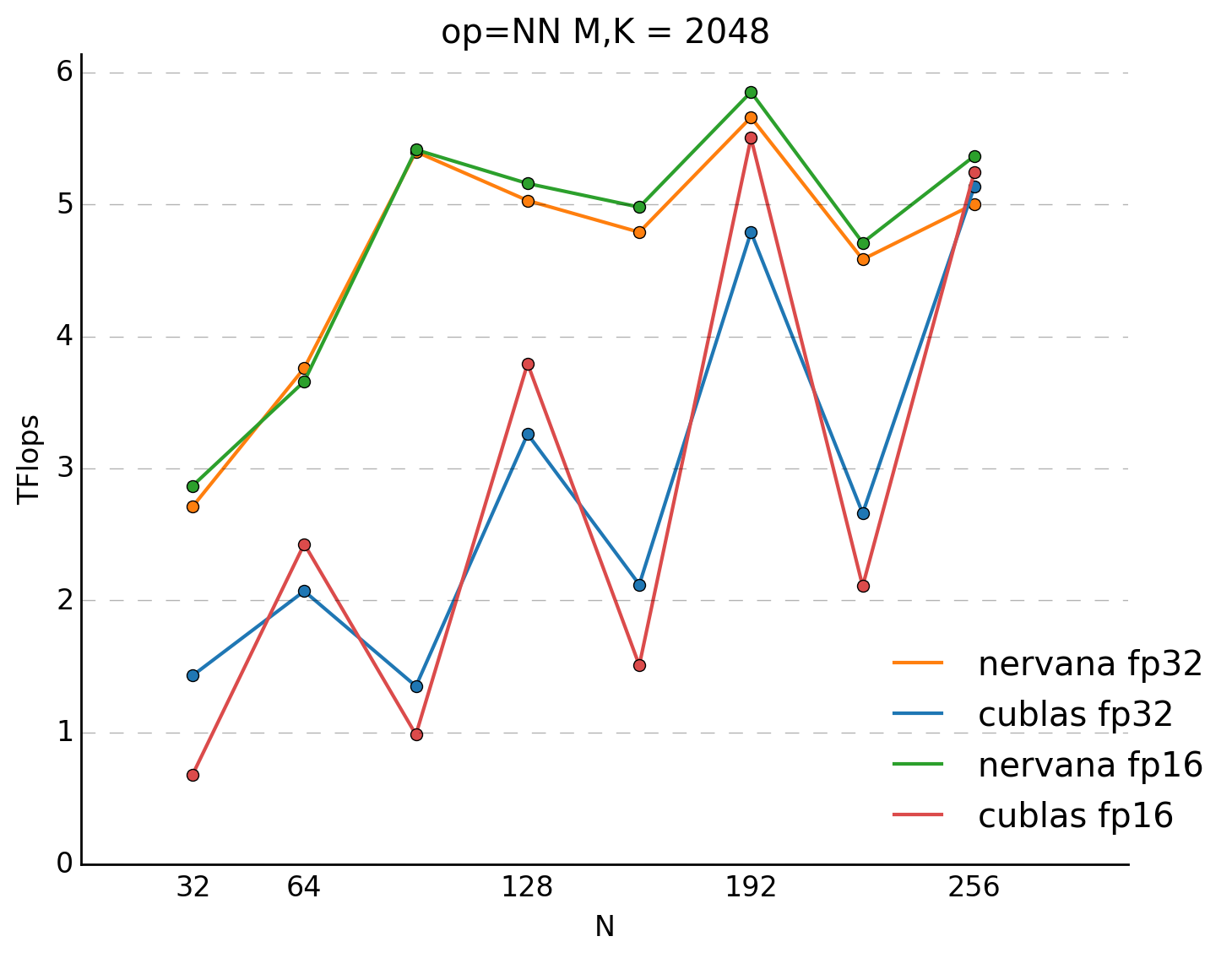# Optimizing RNN performance

Part I: Investigating performance of GPU BLAS Libraries
 Erich ElsenBaidu Silicon Valley AI Lab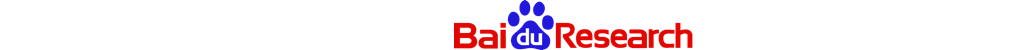## 1. Preamble

This is part I of a multi-part series detailing some of the techniques we've used here at Baidu's Silicon Valley AI Lab to accelerate the training of recurrent neural networks. This part focuses on GEMM performance. Later entries might focus on how we parallelize across GPUs, working with half precision, efficient GPU CTC calculation, GRU and LSTM implementation tricks

## 2. Audience

There are four main groups that this blog post is targeted towards:

2. Researchers using a Deep Learning framework such as Torch7, Theano or Caffe. This post will help you pick appropriate sizes for your layers and mini-batches to achieve optimal performance. Picking incorrectly can slow down your training time by a factor of 4x. It will also help you make sure your RNN implementations are as efficient as possible.

3. The authors of Deep Learning frameworks. There are better options than cuBLAS, and some tricks, that if integrated could result in a large speed increase for all of your users.

4. Low level library implementors. This blog post will detail many of the typical sizes and patterns that are of interest to researchers working with RNNs.

## 3. Introduction

Most researchers engaging in Neural Network research have been using GPUs for training for some time now due to the speed advantage they have over CPUs. GPUs from NVIDIA are almost universally preferred because they come with high quality BLAS (cuBLAS) and convolution (cuDNN) libraries. Achieving optimal performance across a wide range of hardware and input sizes is extremely challenging for library writers and there has been some work outside of NVIDIA on libraries focused on achieving even better performance for problem sizes relevant to deep learning.

Scott Gray of Nervana Systems, has written high performance GEMM and space-domain convolution libraries for Maxwell architecture GPUs which are used in their high performance deep learning framework Neon. Facebook has focused on frequency-domain based techniques for their convolution libraries. We have also written some libraries internally for special cases not covered by any existing libraries.

Here at Baidu's Silicon Valley Artificial Intelligence Lab (SVAIL) we spend a lot of time tackling problems that have sequential data dependencies that are best modeled with Recurrent Neural Networks (RNNs). The cost of evaluating these networks is dominated by matrix-matrix multiplies (the GEMM operation in BLAS terminology).

This is in contrast to Neural Networks that work on images where the data dependencies are hierarchically local and the evaluation cost is almost entirely due to convolutions and related operations.

Most networks consist of a mix of both operations. For example, our speech system has at least one layer of convolution, but the evaluation cost is dominated by the recurrent portions of the network. And image networks have layers that are calculated using matrix multiplies, but they tend to be an insignificant part of the evaluation cost. This paper has a nice accounting of the flops in the various layers of image style convnets. For that reason we'll be focusing on GEMM operations in this blog post about RNN performance.

## 4. Mapping BLAS conventions to NN conventions

Common terminology to describe a matrix problem is the triple (M, N, K), which describes the sizes of the matrices involved, and the “op” which tells us which matrices (if any) are transposed. These two pieces of information combined with the datatype (double, single or half) determine the speed of the operation.When we have a fully connected layer in a NN, we generally care how many units there are at the input and how many units are at the output. Additionally, as part of the optimization we have a mini-batch hyper-parameter. The mapping between these numbers is straightforward:

• M = the number of outputs
• K = the number of inputs
• N = the size of the minibatch

We often ignore the minibatch when writing our algorithms down, since it is technically a choice of the optimization, which is why the typical equation you see for forward prop through a layer looks like a matrix-vector product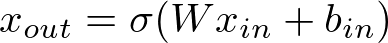. The matrix W is M rows by K columns, the vector-like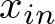is K rows by N columns and the vector-like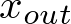is M rows by N columns. The addition of the bias term,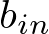, and the evaluation of the non-linearity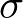have a minor affect on performance in most situations, so we will leave them out of discussions of performance.

## 5. RNN review

There are many introductions and tutorials on the web covering the neat things RNNs can do; one good one is by Andrej Karpathy.

To talk about the performance of RNNs, we just need to look at the equations for going forward and going backward to compute gradients.

The basic equations representing one forward update of a RNN from timestep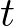to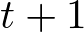look like:

(1)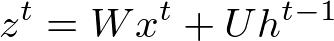(2)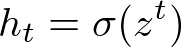where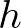is the hidden state of the RNN,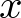is the input from the previous layer,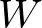is the weight matrix for the input and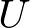is the weight matrix for the recurrent connections.

The hidden weight matrixis necessarily square - the number of hidden units remains the same, so there are the same number of inputs as there are outputs, so M must always equal K. The input weight matrix,does not have to be square - it can connect an arbitrary number of input units to an arbitrary number of hidden units. Often recurrent layers are stacked and in this case the only non-square input matrices will be the one at the very beginning of the recurrent stack and the one at the very end. More complicated versions of RNNs like Gated Recurrent Units (GRUs) and Long Short-Term Memory (LSTMs) have more recurrent weight matrices but they are all also square.

The following picture shows how the forward update looks as multiple steps are chained together.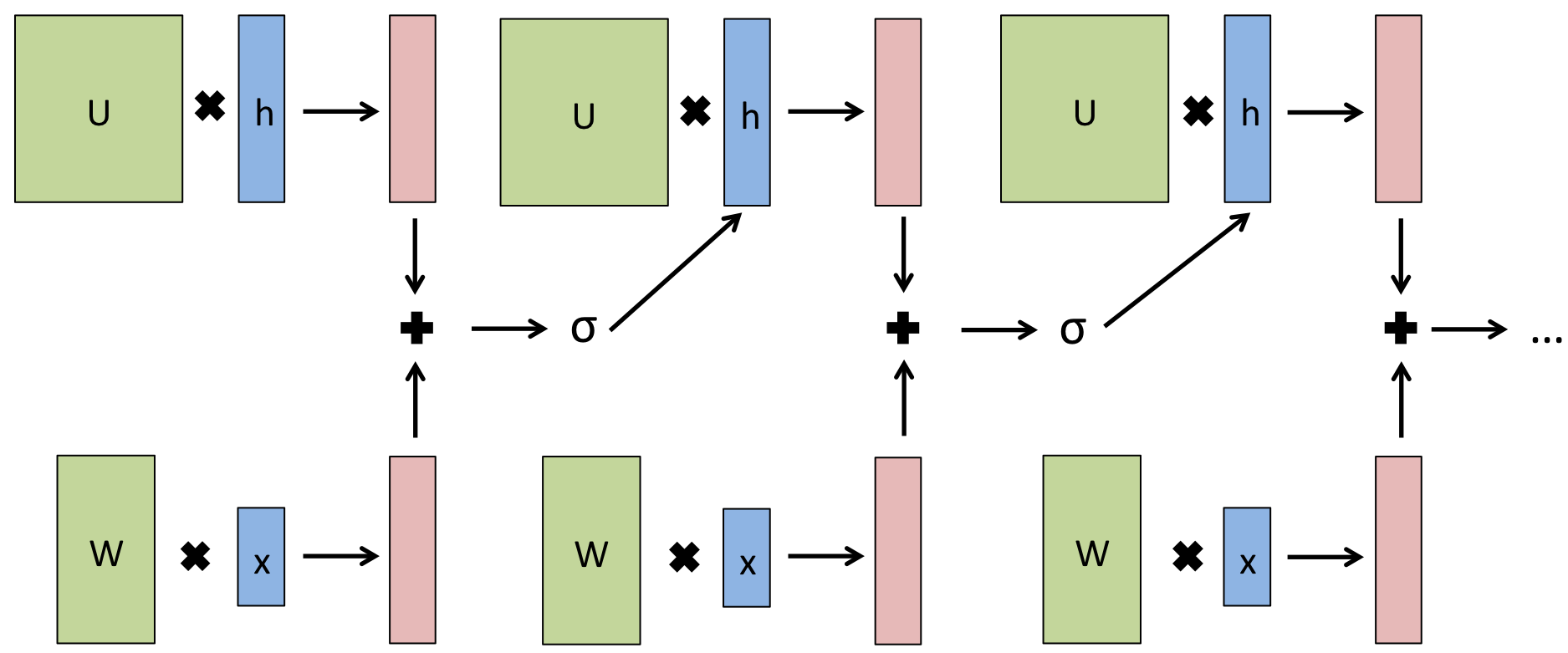To discuss backpropagation, we need to introduce a cost function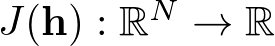. We want to optimize the parameters in our network using an algorithm based on gradient descent, so we will need to find the derivative of this cost with respect to the parametersandof the network, ie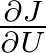and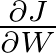.

The way that we usually do the computation is not so obvious if you just start taking derivatives, so for the curious readers, we think that following a derivation of back-propagation for a RNN and seeing how that maps to the computations we perform in practice could be quite useful. If you are interested, check it out here. Otherwise, the final result, is that we need to perform the four steps below, for a sequence with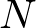time steps.

1. Set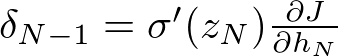2. Calculate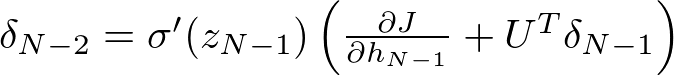3. Repeat 2 until reaching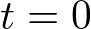.

4. Use equation (3) to get the actual gradients

(3)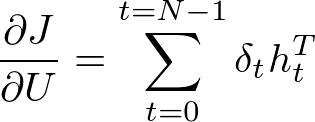The derivative with respect tois exactly the same except for the last step where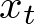replaces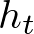(you don't need to recalculate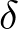).

And finally, if there was another layer below the one that was depicted, then we would need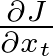, which if you go through a similar derivation, can be shown to be:

(4)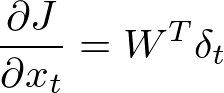In conclusion, the important operations (in terms of performance) will be these three variants of GEMM:

1. the NN op for the forward-pass multiplications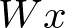and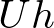.
2. the TN op for the backward-pass multiplications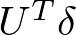and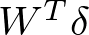3. the NT op for the update calculations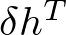and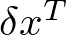### 5.1. Mini-batch

It is widely recognized that increasing the size of a mini-batch is important for decreasing the time to convergence of SGD because the increase in efficiency due to the larger batch size more than compensates for the increase in iterations required to reach a desired level of accuracy. This is true up to a certain point, which we have found to be at least a mini-batch of 512 in our system. Different networks, solving different problems with different data may see different results.

Unfortunately, the performance of GEMM libraries is not monotonically increasing with batch size as our mental model might indicate. There are hardware constraints and implementation choices that favor some sizes over others. It is important to examine the actual performance curves and choose batch sizes that yield the best performance.

Mini-batches are usually grouped by sequence length when training on problems with variable length sequences to avoid wasted work computing time steps that are valid for only a small number of elements in a mini-batch.

The upper bound on the size of a mini-batch is determined by the length of sequences in the training data, the available memory, the implementation of the memory allocator, and the data type used to store activations. The activationsandmust be saved for each time step of the RNN, so longer sequences require more memory. If the memory allocator is implemented as raw calls to cudaMalloc, then the mini-batch is constrained by the length of the longest sequence. Schemes with variable size mini-batches are possible, with smaller mini-batches for long sequences, but this is rarely done in practice. Custom memory allocators which can fall back to host-paged memory (memory that is on the host, but addressable from the GPU) for the longest sequences can allow sequences of essentially unlimited length to be trained at significant speed penalty for the sequences which are too long for GPU memory and fall back to host memory. This scheme makes the practical limitation on mini-batch defined by the 95th to 99th percentile of sequence lengths.

The above considerations mean that, in practice, mini-batches (per GPU) during training tend to be between 32 and 128. Use of half precision can extend the upper bound to 256. Common sizes for the number of hidden units in the recurrent layer range from 512 up to 2560, so the matrix multiplies that are important for RNN evaluation will be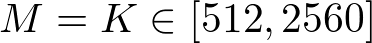and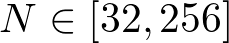for all ops.

## 6. Performance and Strategies for Improvement

All timings were done on a TitanX with GPUBoost disabled. This means the maximum performance of the card is 6.14TFlops - there are 3072 cores, each capable of 1 fused multiply-add (FMA) per clock, operating at an unboosted frequency of 1GHz. This number (the “speed of light”) serves as a reference guide for the maximum performance a gemm operation could achieve. All measurements were made with CUDA 7.0 and driver version 346.59 with the exception of the cuBLAS fp16 measurements which were made with the CUDA 7.5 beta and driver version 352.07 (the numbers for cuBLAS fp32 did not change significantly). Performance of cuBLAS on Kepler based cards (like the K40 and K80) will be significantly lower (2 to over 4x) compared to the performance of the Nervana kernels on Maxwell.

The Nervana GEMM library which is benchmarked below is available here. Both Python and C bindings are provided. The kernels themselves expect a row major data layout in contrast to cuBLAS, which expects a column major layout. The C interface has a wrapper that will take care of calling the kernels correctly with column major data.

The fp16 measurements are for a pseudo-fp16 operation, where the inputs and outputs are fp16, but all of the operations are performed in fp32. Using fp16 for storage of weights and activations has many advantages, but it can be non-trivial to get RNNs to converge when training with pseudo-fp16 operations. We will cover some relevant techniques in a later blog post.

Some plots leave out the fp16 performance for the sake of clarity when the pattern of performance remains the same.

### 6.1. Baseline performance

Assuming we implement our RNN exactly as we described it in the equations above we would get performance something like this: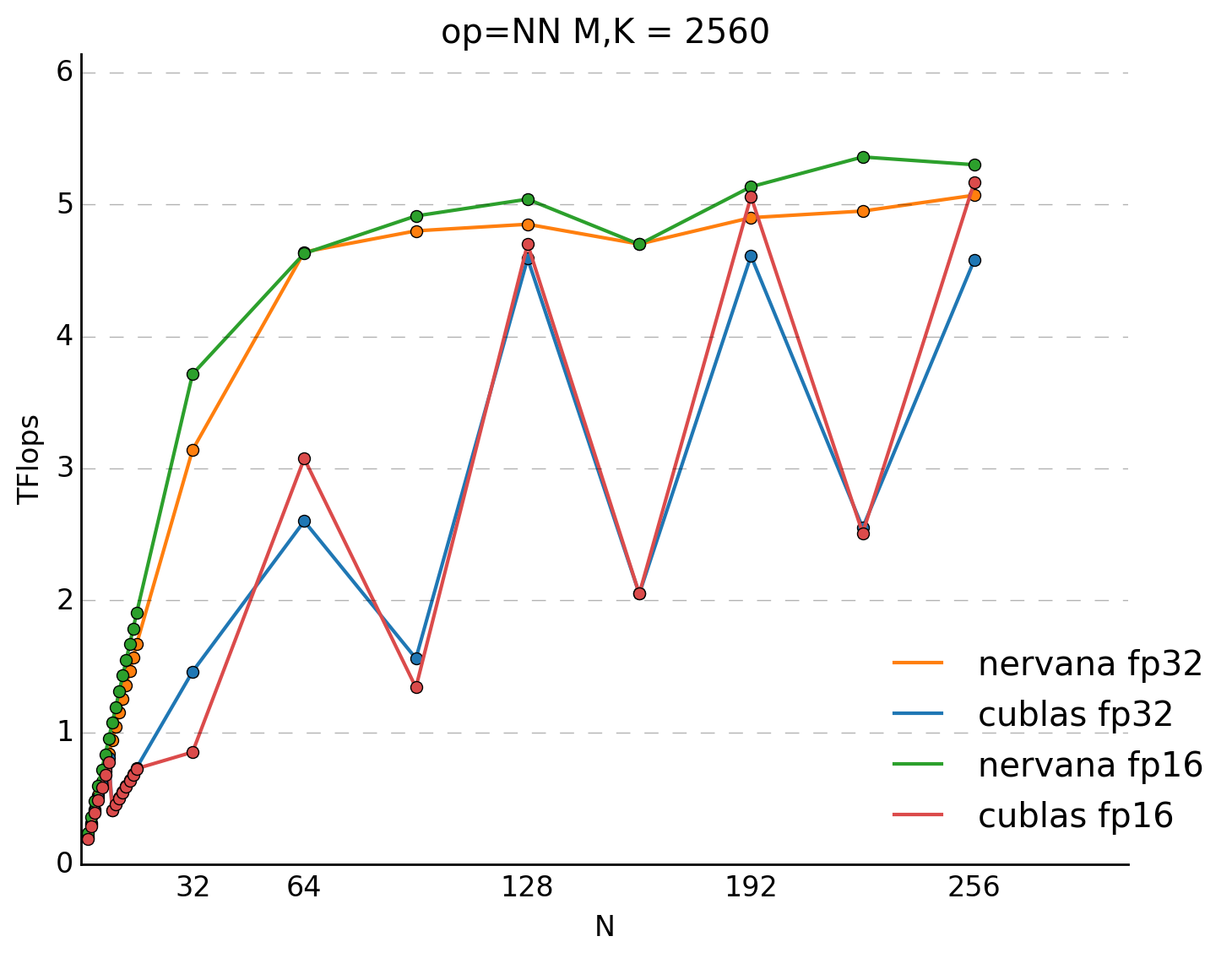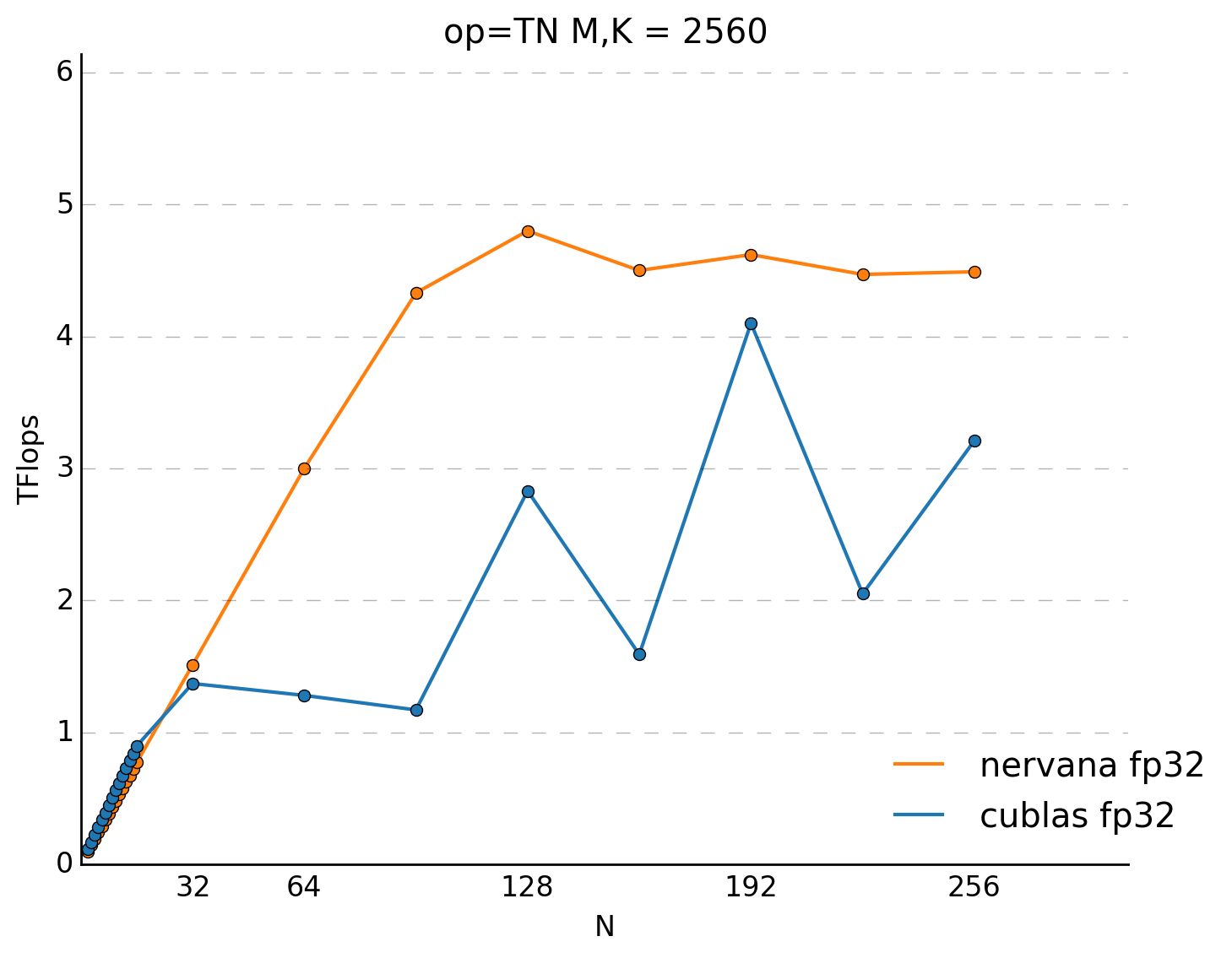We can see the clear advantage of getting to at least a mini-batch of 32, as performance increases by 14x with cuBLAS over a mini-batch of 1 and an amazing, nearly linear increase, of almost 30x for the Nervana kernels. cuBLAS has an unexpected performance regression going from a mini-batch of 8 to 9. It is impressive that the performance of the Nervana kernels is almost monotonically increasing with mini-batch size - matching the mental model of most users. Most users would not expect the oscillations present in the cuBLAS performance and avoid poorly performing sizes.